MATLAB SHEET # 1

Evaluation of integrals can be performed by typing  MATLAB command

syms x; int(f(x))

then pressing enter.

Here f(x) is to be inserted in MATLAB format.  For example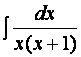will be entered as

syms x; int(1/(x*(x+1))).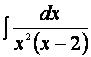will be entered as

syms x; int(1/(x^2*(x-2)))

Practice Problems

Integrate using above MATLAB command.

1)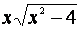2)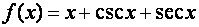3)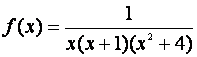4)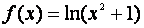(Note: ln function will be entered as log in MATLAB).

5)  Problems 6, 7, 9, 10 of Problems (1.2). Also verify you integration after separating variables in HW problem of (1.4)